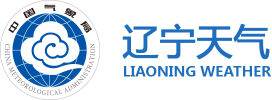14:40实况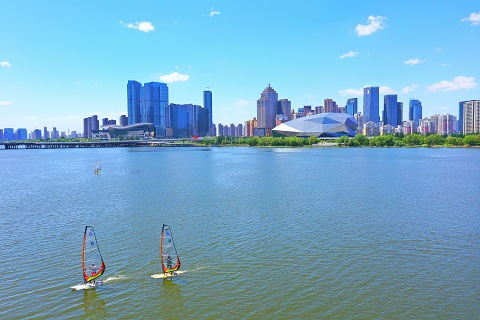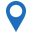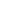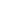•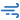•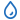•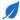•紫外线指数

•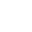穿衣指数

•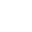洗车指数

•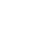感冒指数

• 08时0.1mm
南风
< 3级
• 08时0.1mm
南风
< 3级
• 08时0.1mm
南风
< 3级
• 08时0.1mm
南风
< 3级
• 08时0.1mm
南风
< 3级
• 08时0.1mm
南风
< 3级
• 08时0.1mm
南风
< 3级
• 08时0.1mm
南风
< 3级

• 沈阳城区
• 浑南
• 辽中
• 康平
• 法库
• 新民
• 和平
• 沈河
• 大东
• 皇姑
• 铁西
• 苏家屯
• 沈北新区
• 于洪

• 大连城区
• 瓦房店
• 金州
• 普兰店
• 旅顺
• 长海
• 庄河
• 中山
• 西岗
• 沙河口
• 甘井子

• 鞍山城区
• 台安
• 岫岩
• 海城
• 铁东
• 铁西
• 立山
• 千山

• 抚顺城区
• 新宾
• 清原
• 新抚
• 东洲
• 望花
• 顺城

• 本溪城区
• 本溪县
• 平山
• 桓仁
• 溪湖
• 明山
• 南芬

• 丹东城区
• 凤城
• 宽甸
• 东港
• 元宝
• 振兴
• 振安

• 锦州城区
• 凌海
• 古塔
• 义县
• 黑山
• 北镇
• 凌河
• 太和

• 营口城区
• 大石桥
• 盖州
• 站前
• 西市
• 鲅鱼圈
• 老边

• 阜新城区
• 彰武
• 海州
• 新邱
• 太平
• 清河门
• 细河

• 辽阳城区
• 辽阳县
• 灯塔
• 弓长岭
• 白塔
• 文圣
• 宏伟
• 太子河

• 铁岭城区
• 开原
• 昌图
• 西丰
• 调兵山
• 银州
• 清河

• 朝阳城区
• 双塔
• 凌源
• 喀左
• 北票
• 龙城
• 建平县

• 盘锦城区
• 大洼
• 盘山
• 双台子
• 兴隆台

• 葫芦岛城区
• 建昌
• 绥中
• 兴城
• 连山
• 龙港
• 南票